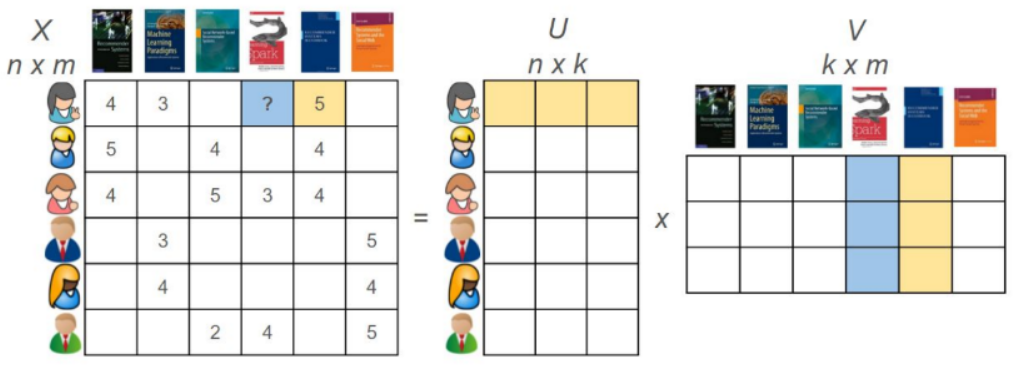## Implicit Introduction

Implicit is an open source collaborative filtering project that contains a variety of popular recommendation algorithms, with the main application scenario being recommendations for implicit feedback behaviors. The main algorithms included are.

• ALS (alternating least squares)
• BRP (Bayesian Personalized Ranking)
• Logistic Matrix Factorization
• Nearest neighbor model using Cosine, TF-IDF or BM25## Implicit use

Data preparation

Implicit input needs to use the data format of user_id/item_id/rating, where for implicit rating scenarios, it can be set according to the specific situation, for example.

• Set different rating according to browsing time
• Different ratings according to the depth of browsing (whether or not you have seen the images, reviews, etc.)
• Set different ratings according to different behaviors (browsing, favorites, adds)

Model training

 `````` 1 2 3 4 5 6 7 8 9 10 11 12 13 14 15 16 17 18 19 20 21 `````` ``````import pandas as pd import numpy as np import scipy.sparse as sparse import implicit df = pd.read_csv("./data/user_visit.csv") df['user_label'], user_idx = pd.factorize(df['user_id ']) df['item_label'], item_idx = pd.factorize(df['item_id ']) sparse_item_user = sparse.csr_matrix((df['rating'].astype(float), (df['item_label'], df['user_label']))) sparse_user_item = sparse.csr_matrix((df['rating'].astype(float), (df['user_label'], df['item_label']))) model = implicit.als.AlternatingLeastSquares(factors=50, regularization=0.1, iterations=50) model.fit(sparse_item_user) data = { 'model.item_factors': model.item_factors, 'model.user_factors': model.user_factors, 'item_labels': item_idx, } als_model_file = "user_visit.npz" np.savez(als_model_file, **data) ``````

Notes.

• The ALS algorithm is used here, and there is no good solution for how to tune the specific model parameters, and the parameters given are given arbitrarily.
• Here the model results are stored in the .npz file, which is easy to use directly at a later stage, instead of being trained every time it is used.
• Need to recode the original user_id, item_id, otherwise it will report an error

Model use

 `````` 1 2 3 4 5 6 7 8 9 10 11 12 13 14 15 16 17 18 19 20 21 22 `````` ``````# 加载模型 data = np.load(als_model_file, allow_pickle=True) model = implicit.als.AlternatingLeastSquares(factors=data['model.item_factors'].shape) model.item_factors = data['model.item_factors'] model.user_factors = data['model.user_factors'] model._YtY = model.item_factors.T.dot(model.item_factors) item_labels = data['item_labels'] # 基于酒店推荐： item_id= 1024 item_lable = list(item_labels).index(item_id) related = model.similar_items(item_lable, N=10) for item_lable, score in related: print(item_labels[item_lable], score) # 基于用户推荐 user_id = 10 user_label = list(user_idx).index(user_id) sparse_user_items = sparse_item_user.T.tocsr() recommendations = model.recommend(user_label, sparse_user_items) for item_id, score in recommendations: print(item_idx[item_id], score) ``````

Recommendation in real time

The real-time recommendation solution uses an offline model combined with real-time behavior to make recommendations, rather than deploying the entire model to run online in real-time. The main difference in the middle is that the user ID does not exist, so direct recommendations cannot be made using the userid. The specific implementation is as follows.

 `````` 1 2 3 4 5 6 7 8 9 10 11 12 13 14 15 `````` ``````item_ids = [1024,2046] item_weights = [2,3] user_label = 0 user_items = None item_lb = [list(item_labels).index(i) for i in item_ids] user_ll =  * len(item_ids) confidence =  * len(item_ids) if item_weights is None else item_weights user_items = sparse.csr_matrix((confidence, (user_ll, item_lb))) recommendations = model.recommend(user_label, user_items, N=10, recalculate_user=True) for item_id, score in recommendations: print(item_labels[item_id], score) #根据返回的结果，获取推荐理由： itemid = list(item_labels).index(2048) model.explain(user_label, user_items, itemid, user_weights=None, N=1) ``````

Reference.